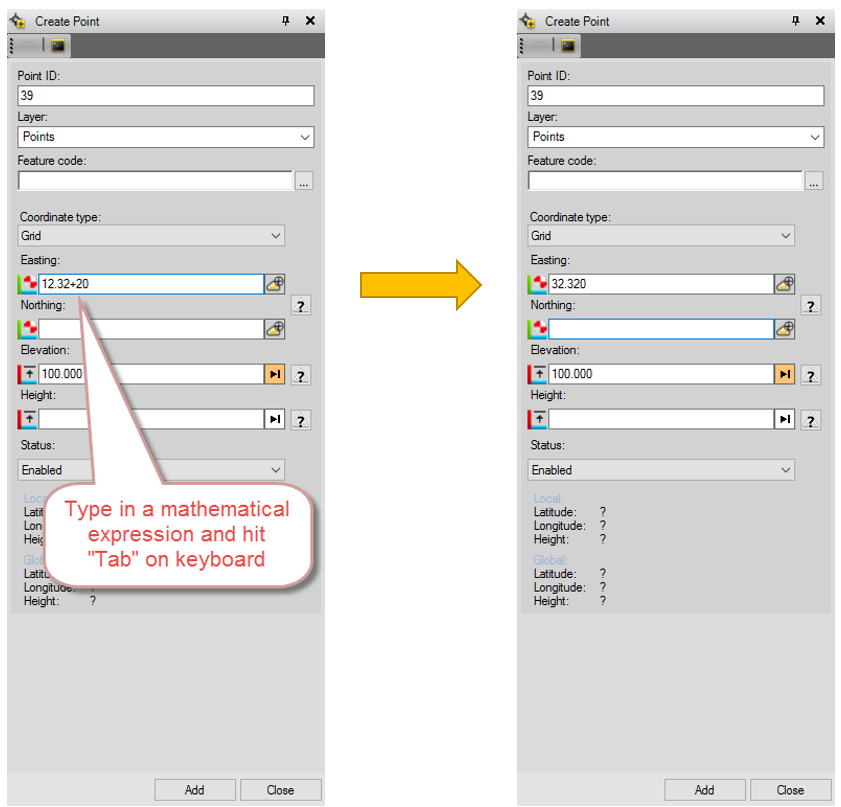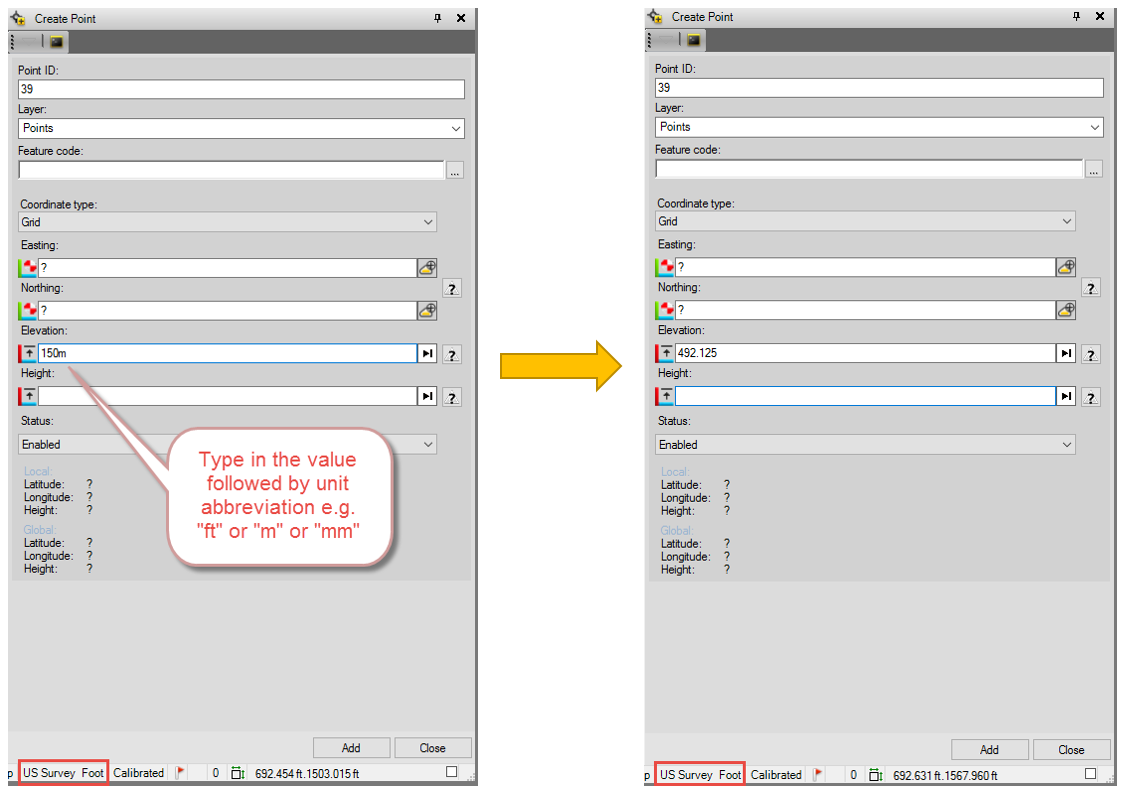# Blogs

## Tip of the Week #8:  Doing Math in TBC commands

####By Boris Skopljak posted 05-02-2017 13:46

Did you know you can use TBC as calculator? Yes, that’s right. You don’t have to use your calculator if you are doing some simple math like  adding or subtracting values before you key them in TBC or computing units.

First, open any command in TBC that expect some input e.g. Create Points, or Create Polyline and click in the coordinate field. You can see in the image below where we used “12.32+20” as an example and after hitting “Tab” got “32.320”.You can do mathematical expression of addition, subtraction, division and multiplication (+, -, *, /). You can have multiple numbers e.g. “12+4+6” to get “22” or you can use “12+4*2” to get “20” (yes, we know the priority of operations :)).Note that you cannot use spaces “ “ between the number and mathematical operators. The numbers provided will be in project units.

If you have a units on paper in metric and your project is in survey feet or vice versa, you can do that as well. Let’s take an example of a project where you have setup of survey feet and you are keying in a value of 150m (meters).

Open a command e.g. Create Point and in the elevation field type in “150m” and hit “Tab”. You can use standard abbreviations for distance units like “mm, cm, m, km, ft”.We hope this tip saves you few minutes on your daily work by not having to open a calculator to do some quick calculations.

Happy Surveying,

TBC Team